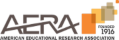Paper Summary
Share...

### Using Worked Examples to Improve Middle School Students' Fraction Knowledge

Mon, April 20, 8:15 to 10:15am, Virtual Room

#### Abstract

It is well-established that students have difficulty learning fractions (e.g., Sophian, 2007), which may be particularly problematic given that a foundational knowledge of fractions (both concepts and procedures) is critical for success in learning algebra (Brown & Quinn, 2007; NMAP, 2008; Siegler et al., 2012; Booth, Newton, & Twiss-Garrity, 2014). Improving fraction knowledge may thus be crucial for students’ continued learning in mathematics. The purpose of the present study was to investigate whether applying a proven instructional technique—explaining worked examples—can remediate different facets of students’ fraction knowledge. The technique of having students explain correct and incorrect solutions has been successfully used to enhance learning of computational procedures in a wide variety of mathematical domains (e.g., Sweller & Cooper, 1985; Paas & Van Merriënboer, 1994). Importantly, however, it can also be used to support conceptual understanding of non-computational content (Booth, Oyer et al., 2015; Davenport et al., 2013). This suggests that the same technique can be used successfully on both computation-based items (e.g., examples showing correct and incorrect ways to solve fraction arithmetic problems) and magnitude-based items (e.g., examples showing correct and incorrect ways to think about fractions as numbers).

In the present study, 91 eighth-grade students completed 8 assignments with problems focused on computational and magnitude knowledge of fractions. Control assignments contained 8 practice problems for students to solve. In the experimental versions of the assignments, half of the practice problems were replaced with correct or incorrect examples of solutions to the problems for students to explain. Students (within classrooms) were randomly assigned to one of three conditions: 1) Control (practice only), 2) Experimental assignments for computation topics and control assignments for magnitude topics, or 3) Experimental assignments for both computation and magnitude topics. Students were also tested on their fraction computation (e.g., 2/3 + 7/10; 6/7 x 3/5) and fraction magnitude knowledge (estimating the placement of fractions on a number line with endpoints of 0 and 1) at the beginning of the school year, after completing the assignments, and at the end of the school year. Results indicate that, across the school year, students increased the percentage of items on the fraction computation test solved correctly and decreased their average percent absolute error (PAE) on their fraction magnitude estimates. Students who received worked examples for the magnitude problems improved more than students in the other two conditions on PAE for their fraction number line estimates; however, improvements in fraction computation scores were not dependent on receiving worked examples on the computation problems.

Implications from these results include the first evidence that having students explain correct and incorrect worked examples can improve their knowledge of the magnitudes of numbers; further research is needed to determine whether these improvements yield subsequent gains in algebra knowledge and learning. Future studies should also investigate whether worked examples are indeed not effective for helping students with fraction computation procedures, or if fraction computation worked examples might have an impact when students are first learning fraction computation procedures earlier in middle school.